# CHAPTER 6 6 3 The Central Limit Theorem

• Slides: 10CHAPTER 6 6 -3 The Central Limit Theorem. Instructor: Alaa saud Note: This Power. Point is only a summary and your main source should be the book.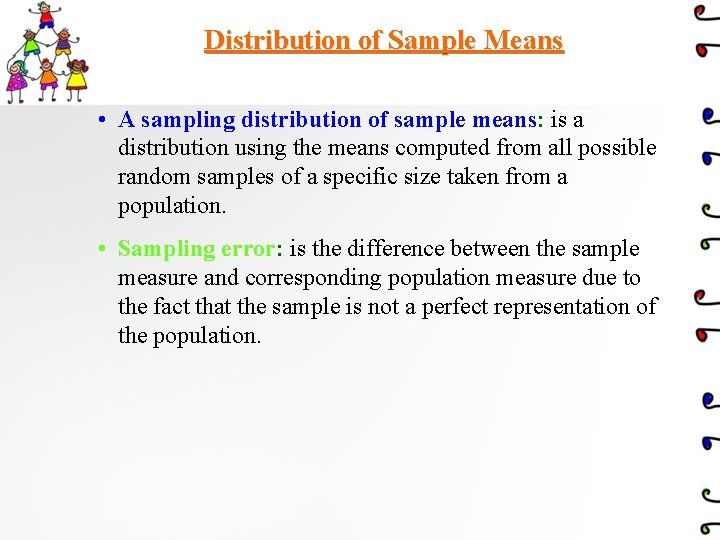Distribution of Sample Means • A sampling distribution of sample means: is a distribution using the means computed from all possible random samples of a specific size taken from a population. • Sampling error: is the difference between the sample measure and corresponding population measure due to the fact that the sample is not a perfect representation of the population.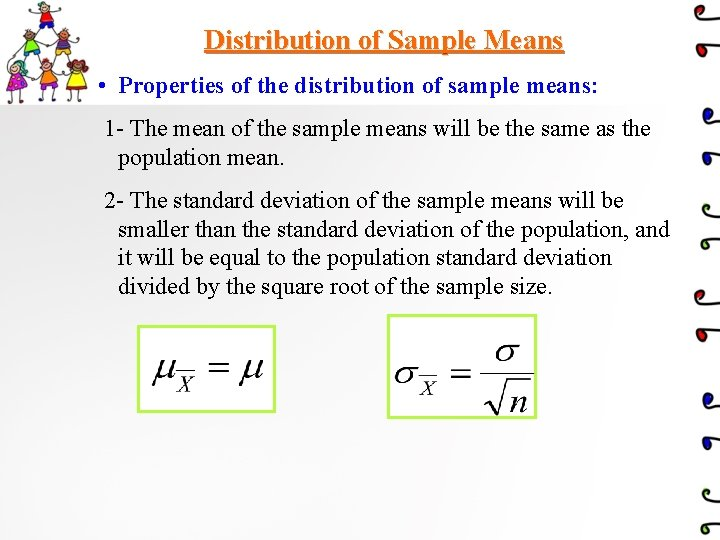Distribution of Sample Means • Properties of the distribution of sample means: 1 - The mean of the sample means will be the same as the population mean. 2 - The standard deviation of the sample means will be smaller than the standard deviation of the population, and it will be equal to the population standard deviation divided by the square root of the sample size.• The Central Limit Theorem: • As the sample size n increase without limit, the shape of the distribution of the sample means taken with replacement from a population with mean and standard deviation will approach a normal distribution. This distribution will have a mean and a standard deviation • The formula for z values is:Two important things when we use Central limit theorem : 1 - when the original variable is normally distributed , the distribution of the sample means will be normally distributed , for any sample size n. 2 - when the original variable might not be normally distributed, a sample size must be 30 or more to approximate the distribution of sample means to normal distribution.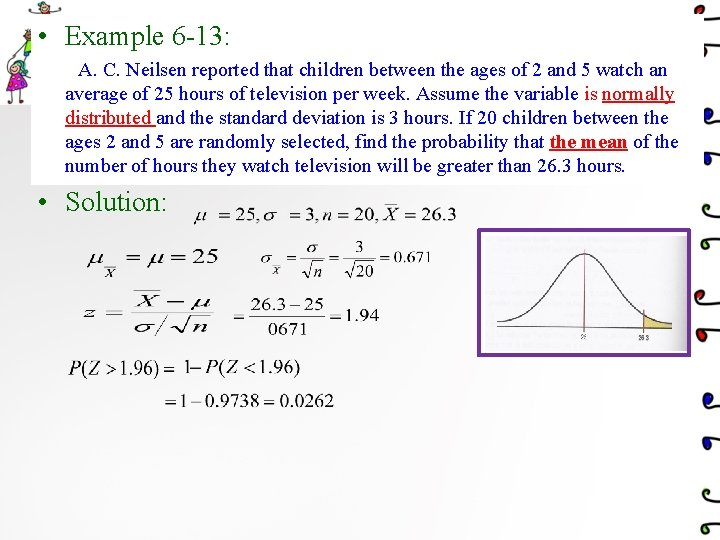• Example 6 -13: A. C. Neilsen reported that children between the ages of 2 and 5 watch an average of 25 hours of television per week. Assume the variable is normally distributed and the standard deviation is 3 hours. If 20 children between the ages 2 and 5 are randomly selected, find the probability that the mean of the number of hours they watch television will be greater than 26. 3 hours. • Solution: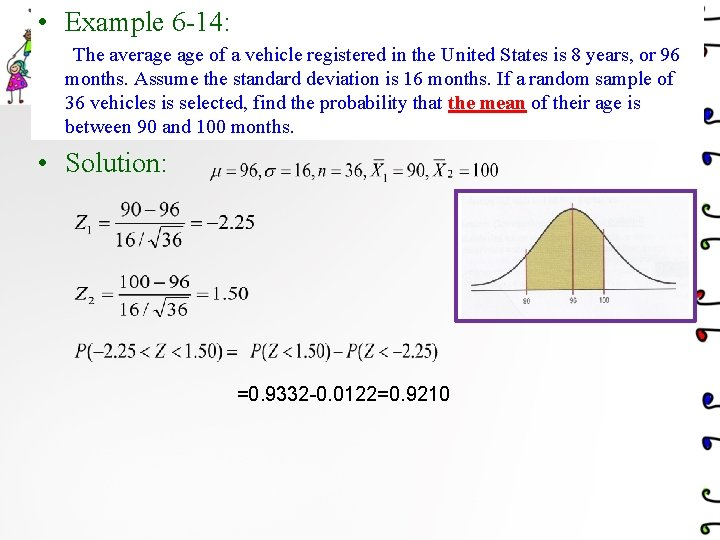• Example 6 -14: The average of a vehicle registered in the United States is 8 years, or 96 months. Assume the standard deviation is 16 months. If a random sample of 36 vehicles is selected, find the probability that the mean of their age is between 90 and 100 months. • Solution: =0. 9332 -0. 0122=0. 9210• Example 6 -15: The average number of pounds of meat that a person consumes per year is 218. 4 pounds. Assume that the standard deviation is 25 pounds and the distribution is approximately normal. a. Find the probability that a person selected at random consumes less than 224 pounds per year. b. If a sample of 40 individuals is selected, find the probability that the mean of the sample will be less than 224 pounds per year. • Solution: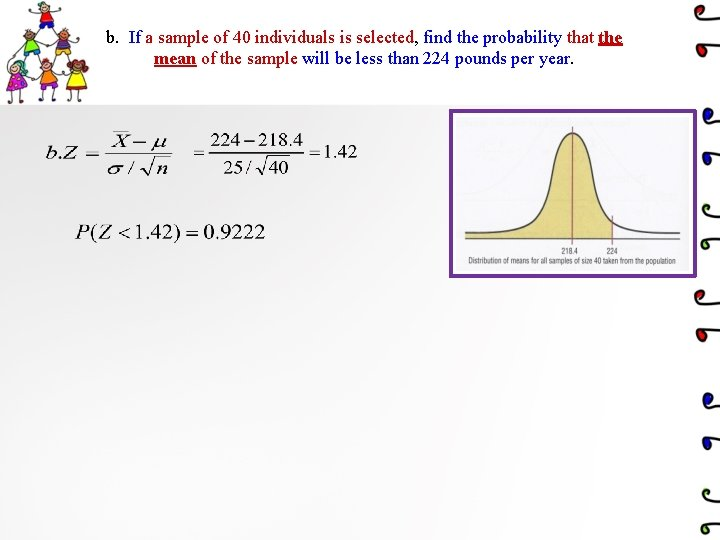b. If a sample of 40 individuals is selected, find the probability that the mean of the sample will be less than 224 pounds per year.Note: This Power. Point is only a summary and your main source should be the book.